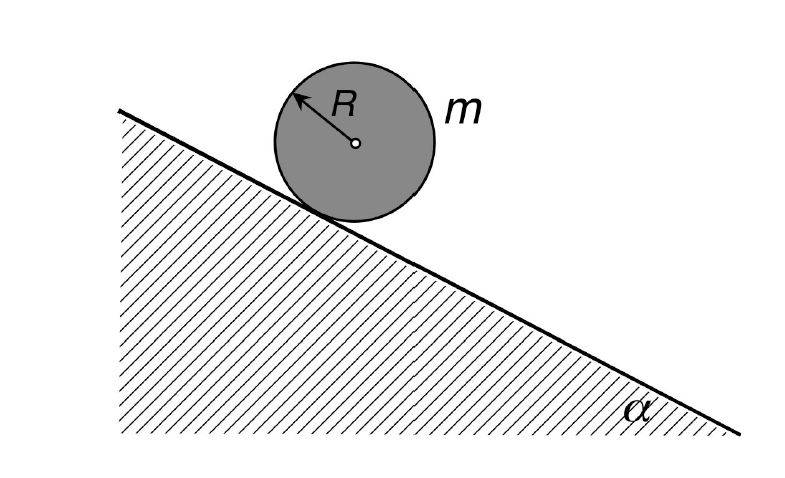# Cylinder rolling down the hill

• RiotRick

## Homework StatementA solid cylinder rolls down an inclined plane because of stiction. How does angular velocity change over time?
Given:
##m,R,g,\alpha,\mu## where ##\mu## is the friction coefficient

## Homework Equations

[/B]
##J_{cm} = 1/2 m*r^2## moment of inertia
m = mass of the cylinder
Parallel Axis theorem: ##J=J_{cm}+mr^2##
## M = I*d\omega/dt ## = Torque

## The Attempt at a Solution

First I thought I take the torque at the center of mass pulling it down
##R*mg*sin(\alpha)=d\omega/dt*(J_{cm}+mr^2)##
which gives me as an end result:
## d\omega/dt = \frac{2*g*sin(\alpha)}{3*r} ##
= ## \omega = \frac{2*g*sin(\alpha)}{3*r}*t ##
Then I thought it would have been simpler if I looked at the point of friction:
## M/J = r \times F / J = r*F_{friction}/J = \mu*r*mg*cos(\alpha)/J=d\omega/dt ##
=## \frac{2*\mu*g*cos(\alpha)}{3r}=d\omega/dt ##
=## \frac{2*\mu*g*cos(\alpha)}{3r}*t=\omega ##

Are both correct, one of them or both garbage?

#### Attachments

You cannot just ignore the change in linear velocity.

Edit: Also, is the cylinder assumed to be rolling without slipping or do you need to take slipping into account?

First I thought I take the torque at the center of mass pulling it down
It does not seem to me that is what you did. You appear to have taken moments about the point of contact. In particular, you had to use the parallel axis theorem to get the MoI.
This is a good approach because it avoids dealing with the frictional force.
Then I thought it would have been simpler if I looked at the point of friction:
Which is what I think you did the first time. If you mean to consider the torque exerted by friction then you would now be taking moments about the mass centre, so the MoI is just ½mr2, but somehow you still got a 3 in there.
You will need another equation relating the frictional force to the linear acceleration, and another relating rotational to linear acceleration when rolling.
Also, you seem to have applied this popular but erroneous equation:
Static frictional force = coefficient of static friction x normal force.
That contains a serious flaw. Can you spot it?
is the cylinder assumed to be rolling without slipping or do you need to take slipping into account?
It says "stiction", which I take to mean static friction.

Thank you both for the quick answer. It's rolling without slipping

@haruspex: So you think the first answer is correct?
I used in both cases the parallel axis theorem. So you mean in the 2nd case it's just the moment of inertia? Why is that? Because the rotation axis never falls together with the "ground"?

That contains a serious flaw. Can you spot it?
No. I've never heard that there is something fishy with it

No. I've never heard that there is something fishy with it
The coefficient of static friction tells you how hard you can push something before it "breaks loose" and starts slipping.

Say, for instance, you have a 50 kg box on a floor with a coefficient of static friction of 0.1 and gravity of 10 meters/sec^2. You rapidly calculate that you need a horizontal force of 50 * 0.1 * 10 = 50 N to overcome static friction and get it moving.

What is the force of static friction if you push the box horizontally with only 40 N?

in the 2nd case it's just the moment of inertia?
If you are taking moments about the mass centre then the torque you calculate is producing an angular acceleration about the mass centre. So no parallel axis involved.